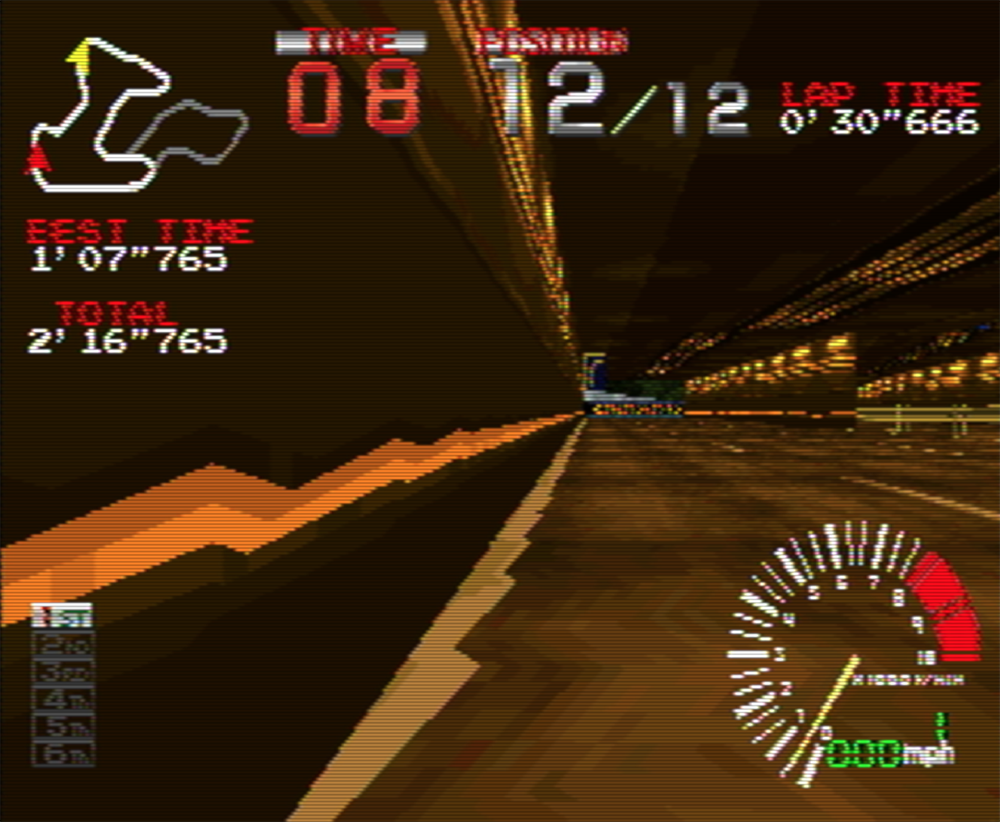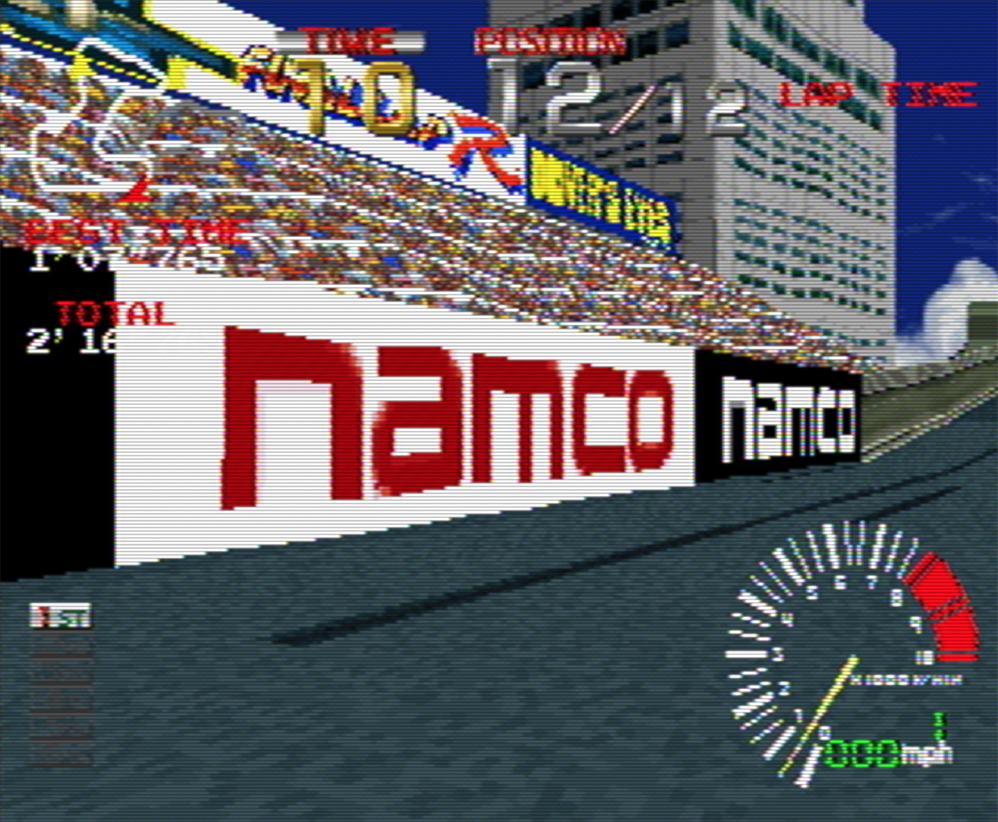# WebGL2Fundamentals.org

Fix, Fork, Contribute

# WebGL2 3D Perspective Correct Texture Mapping

This post is a continuation of a series of posts about WebGL. The first started with fundamentals. This article covers perspective correct texture mapping. To understand it you probably need to read up on perspective projection and maybe texturing as well. You also need to know about varyings and what they do but I'll cover them briefly here.

So in the "how it works" article we covered how varyings work. A vertex shader can declare a varying and set it to some value. Once the vertex shader has been called 3 times WebGL will draw a triangle. While it's drawing that triangle for every pixel it will call our fragment shader and ask it what color to make that pixel. Between the 3 vertices of the triangle it will pass us our varyings interpolated between the 3 values.

v_color is interpolated between v0, v1 and v2

Going back to our first article we drew a triangle in clip space, no math. We just passed in some clip space coordinates to a simple vertex shader that looked like this

``````  #version 300 es

// an attribute is an input (in) to a vertex shader.
// It will receive data from a buffer
in vec4 a_position;

// all shaders have a main function
void main() {

// gl_Position is a special variable a vertex shader
// is responsible for setting
gl_Position = a_position;
}
``````

``````  #version 300 es

// fragment shaders don't have a default precision so we need
// to pick one. highp is a good default
precision highp float;

// we need to declare an output for the fragment shader
out vec4 outColor;

void main() {
// Just set the output to a constant reddish-purple
outColor = vec4(1, 0, 0.5, 1);
}
``````

So let's make that draw 2 rectangles in clip space. We'll pass it this data with `X`, `Y`, `Z`, and `W` for each vertex.

``````var positions = [
-.8, -.8, 0, 1,  // 1st rect 1st triangle
.8, -.8, 0, 1,
-.8, -.2, 0, 1,
-.8, -.2, 0, 1,  // 1st rect 2nd triangle
.8, -.8, 0, 1,
.8, -.2, 0, 1,

-.8,  .2, 0, 1,  // 2nd rect 1st triangle
.8,  .2, 0, 1,
-.8,  .8, 0, 1,
-.8,  .8, 0, 1,  // 2nd rect 2nd triangle
.8,  .2, 0, 1,
.8,  .8, 0, 1,
];
``````

Here's that

Let's add a single varying float. We'll pass that directly from the vertex shader to the fragment shader.

``````  #version 300 es

in vec4 a_position;
+  in float a_brightness;

+  out float v_brightness;

void main() {
gl_Position = a_position;

+    // just pass the brightness on to the fragment shader
+    v_brightness = a_brightness;
}
``````

In the fragment shader we'll use that varying to set the color

``````  #version 300 es

precision highp float;

+  // passed in from the vertex shader and interpolated
+  in float v_brightness;

// we need to declare an output for the fragment shader
out vec4 outColor;

void main() {
*    outColor = vec4(v_brightness, 0, 0, 1);  // reds
}
``````

We need to supply data for that varying so we'll make a buffer and put in some data. One value per vertex. We'll set all the brightness values for vertices on the left to 0 and those on the right to 1.

``````  // Create a buffer and put 12 brightness values in it
var brightnessBuffer = gl.createBuffer();

// Bind it to ARRAY_BUFFER (think of it as ARRAY_BUFFER = brightnessBuffer)
gl.bindBuffer(gl.ARRAY_BUFFER, brightnessBuffer);

var brightness = [
0,  // 1st rect 1st triangle
1,
0,
0,  // 1st rect 2nd triangle
1,
1,

0,  // 2nd rect 1st triangle
1,
0,
0,  // 2nd rect 2nd triangle
1,
1,
];

gl.bufferData(gl.ARRAY_BUFFER, new Float32Array(brightness), gl.STATIC_DRAW);
``````

We also need to look up the location of the `a_brightness` attribute at init time

``````  // look up where the vertex data needs to go.
var positionAttributeLocation = gl.getAttribLocation(program, "a_position");
+  var brightnessAttributeLocation = gl.getAttribLocation(program, "a_brightness");
``````

and setup that attribute at render time

``````  // Turn on the attribute
gl.enableVertexAttribArray(brightnessAttributeLocation);

// Bind the position buffer.
gl.bindBuffer(gl.ARRAY_BUFFER, brightnessBuffer);

// Tell the attribute how to get data out of brightnessBuffer (ARRAY_BUFFER)
var size = 1;          // 1 component per iteration
var type = gl.FLOAT;   // the data is 32bit floats
var normalize = false; // don't normalize the data
var stride = 0;        // 0 = move forward size * sizeof(type) each iteration to get the next position
var offset = 0;        // start at the beginning of the buffer
gl.vertexAttribPointer(
brightnessAttributeLocation, size, type, normalize, stride, offset);
``````

And now when we render we get two rectangles that are black on the left when `brightness` is 0 and red on the right when `brightness` is 1 and for the area in between `brightness` is interpolated or (varied) as it goes across the triangles.

So then, from the perspective article we know that WebGL takes whatever value we put in `gl_Position` and it divides it by `gl_Position.w`.

In the vertices above we supplied `1` for `W` but since we know WebGL will divide by `W` then we should be able do something like this and get the same result.

``````  var mult = 20;
var positions = [
-.8,  .8, 0, 1,  // 1st rect 1st triangle
.8,  .8, 0, 1,
-.8,  .2, 0, 1,
-.8,  .2, 0, 1,  // 1st rect 2nd triangle
.8,  .8, 0, 1,
.8,  .2, 0, 1,

-.8       , -.2       , 0,    1,  // 2nd rect 1st triangle
.8 * mult, -.2 * mult, 0, mult,
-.8       , -.8       , 0,    1,
-.8       , -.8       , 0,    1,  // 2nd rect 2nd triangle
.8 * mult, -.2 * mult, 0, mult,
.8 * mult, -.8 * mult, 0, mult,
];
``````

Above you can see that for every point on the right in the second rectangle we are multiplying `X` and `Y` by `mult` but, we are also setting `W` to `mult`. Since WebGL will divide by `W` we should get the exact same result right?

Well here's that

Note the 2 rectangles are drawn in the same place they were before. This proves `X * MULT / MULT(W)` is still just `X` and same for `Y`. But, the colors are different. What's going on?

It turns out WebGL uses `W` to implement perspective correct texture mapping or rather to do perspective correct interpolation of varyings.

In fact to make it easier to see let's hack the fragment shader to this

``````outColor = vec4(fract(v_brightness * 10.), 0, 0, 1);  // reds
``````

multiplying `v_brightness` by 10 will make the value go from 0 to 10. `fract` will just keep the fractional part so it will go 0 to 1, 0 to 1, 0 to 1, 10 times.

A linear interpolation from one value to another would be this formula

`````` result = (1 - t) * a + t * b
``````

Where `t` is a value from 0 to 1 representing some position between `a` and `b`. 0 at `a` and 1 at `b`.

For varyings though WebGL uses this formula

`````` result = (1 - t) * a / aW + t * b / bW
-----------------------------
(1 - t) / aW + t / bW
``````

Where `aW` is the `W` that was set on `gl_Position.w` when the varying was as set to `a` and `bW` is the `W` that was set on `gl_Position.w` when the varying was set to `b`.

Why is that important? Well here's a simple textured cube like we ended up with in the article on textures. I've adjusted the UV coordinates to go from 0 to 1 on each side and it's using a 4x4 pixel texture.

Now let's take that example and change the vertex shader so that we divide by `W` ourselves. We just need to add 1 line.

``````#version 300 es

in vec4 a_position;
in vec2 a_texcoord;

uniform mat4 u_matrix;

out vec2 v_texcoord;

void main() {
// Multiply the position by the matrix.
gl_Position = u_matrix * a_position;

+  // Manually divide by W.
+  gl_Position /= gl_Position.w;

// Pass the texcoord to the fragment shader.
v_texcoord = a_texcoord;
}
``````

Dividing by `W` means `gl_Position.w` will end up being 1. `X`, `Y`, and `Z` will come out just like they would if we let WebGL do the division for us. Well here are the results.

We still get a 3D cube but the textures are getting warped. This is because by not passing `W` as it was before WebGL is not able to do perspective correct texture mapping. Or more correctly, WebGL is not able to do perspective correct interpolation of varying.

If you recall `W` was our `Z` value from our perspective matrix). With `W` just being `1` WebGL just ends up doing a linear interpolation. In fact if you take the equation above

`````` result = (1 - t) * a / aW + t * b / bW
-----------------------------
(1 - t) / aW + t / bW
``````

And change all the `W`s to 1s we get

`````` result = (1 - t) * a / 1 + t * b / 1
---------------------------
(1 - t) / 1 + t / 1
``````

Dividing by 1 does nothing so we can simplify to this

`````` result = (1 - t) * a + t * b
-------------------
(1 - t) + t
``````

`(1 - t) + t` when `t` goes from 0 to 1 is the same as `1`. For example if `t` was `.7` we'd get `(1 - .7) + .7` which is `.3 + .7` which is `1`. In other words we can remove the bottom so we're left with

`````` result = (1 - t) * a + t * b
``````

Which the same as the linear interpolation equation above.

Hopefully it's now clear why WebGL uses a 4x4 matrix and 4 value vectors with `X`, `Y`, `Z`, and `W`. `X` and `Y` divided by `W` get a clip space coordinate. `Z` divided by `W` also get a clipspace coordinate in Z and `W` is still used during interpolation of varyings and provides the ability to do perspective correct texture mapping.

### Mid 1990s Game Consoles

As a little piece of trivia the PlayStation 1 and some of the other game consoles from the same era didn't do perspective correct texture mapping. Looking at the results above you can now see why they looked the way they did.• Fundamentals
• WebGL2 vs WebGL1
• Image Processing
• 2D translation, rotation, scale, matrix math
• 3D
• Lighting
• Structure and Organization
• Geometry
• Textures
• Rendering To A Texture